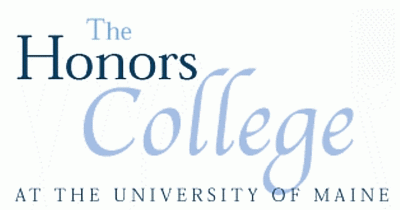### Ode to Applied Physics: The Intellectual Pathway of Differential Equations in Mathematics and Physics Courses: Existing Curriculum and Effective Instructional Strategies

Honors Thesis

Spring 5-2017

#### Abstract

The purpose of this thesis is to develop a relationship between mathematics and physics through differential equations. Beginning with first-order ordinary differential equations, I develop a pathway describing how knowledge of differential equations expands through mathematics and physics disciplines. To accomplish this I interviewed mathematics and physics faculty, inquiring about their utilization of differential equations in their courses or research. Following the interviews I build upon my current knowledge of differential equations in order to reach the varying upper-division differential equation concepts taught in higher-level mathematics and physics courses (e.g., partial differential equations, Bessel equation, Laplace transforms) as gathered from interview responses. The idea is to present a connectedness between the simplest form of the differential equation to the more complicated material in order to further understanding in both mathematics and physics. The main goal is to ensure that physics students aren’t afraid of the mathematics, and that mathematics students aren’t without purpose when solving a differential equation. Findings from research in undergraduate mathematics education and physics education research show that students in physics and mathematics courses struggle with differential equation topics and their applications. I present a virtual map of the various concepts in differential equations. The purpose of this map is to provide a connectedness between complex forms of differential equations to simpler ones in order to improve student understanding and elevate an instructor’s ability to incorporate learning of differential equations in the classroom.